Courses
Courses for Kids
Free study material
Offline Centres
More

# NCERT Exemplar for Class 11 Chemistry Chapter-13 (Book Solutions)Last updated date: 02nd Dec 2023
Total views: 612.3k
Views today: 18.12k

## NCERT Exemplar for Class 11 Chemistry - Hydrocarbons - Free PDF Download

Chapter 13 of class 11 chemistry, HydroCarbons, is all about the various classification of Hydrocarbons. Various uses of hydrocarbons are also discussed in this chapter. The chapter provides the students with a lot of information based on the physical and chemical properties of various kinds of hydrocarbons. Students can download free PDFs of the solutions to all the NCERT exemplar questions given in chapter 13 (Hydrocarbons) from the official website of Vedantu, which they can access at any time.

Free PDF download of NCERT Exemplar for Class 11 Chemistry Chapter 13 - Hydrocarbons solved by expert Chemistry teachers on Vedantu.com as per NCERT (CBSE) Book guidelines. All Chapter 13 - Hydrocarbons exercise questions with solutions to help you to revise the complete syllabus and score more marks in your examinations.

## NCERT Exemplar Solutions For Grade 11 Chemistry Chapter 13 – Hydrocarbons

### Multiple Choice Questions (Type-I)

1. Arrange the following in decreasing order of their boiling points.

(A) n-butane                          (B) 2–methyl butane

(C) n-pentane                         (D) 2, 2–dimethylpropane

(i) A > B > C > D

(ii) B > C > D > A

(iii) D > C > B > A

(iv) C > B > D > A

Ans: (iv) C > B > D > A

The boiling point increases with the increase in the length of the side chain. However, with the branching, the boiling point decreases due to the decrease in the surface area.

2. Arrange the halogens F2, Cl2, Br2, I2, in order of their increasing reactivity with alkanes.

(i) I2< Br2< Cl2< F2

(ii) Br2< Cl2< F2< I2

(iii) F2< Cl2< Br2< I2

(iv) Br2< I2 < Cl2< F2

Ans: (i) I2< Br2< Cl2< F2

The reactivity of halogens with alkanes depends on the electronegativity i.e. higher the electronegativity higher would be the reaction. As down the group reactivity decrease so thus its reactivity.

3. The increasing order of reduction of alkyl halides with zinc and dilute HCl is

(i) R–Cl< R–I < R–Br

(ii) R–Cl< R–Br < R–I

(iii) R–I < R–Br < R–Cl

(iv) R–Br< R–I < R–Cl

Ans: (ii) R–Cl< R–Br < R–I

The reduction of alkyl halides depends upon the C-X bond strength i.e. lesser the bond strength higher will be the reactivity of alkyl halide. As down the group size of the halogens increases thus the bond strength decreases.

4. The correct IUPAC name of the following alkane is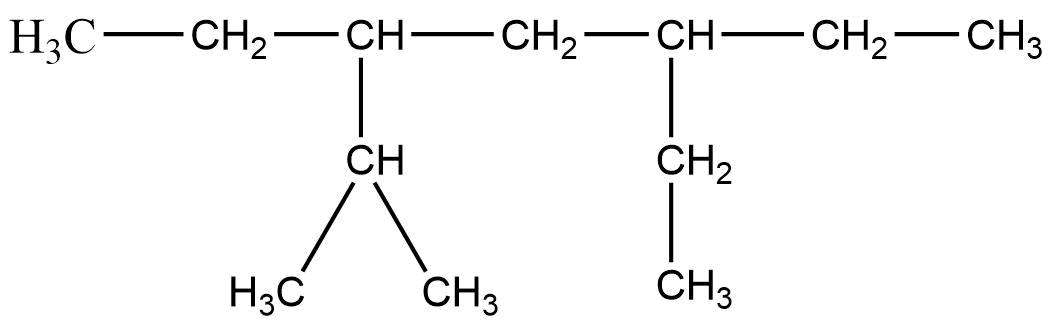(i) 3, 6 – Diethyl – 2 – methyl octane

(ii) 5 – Isopropyl – 3 – ethyl octane

(iii) 3 – Ethyl – 5 – isopropyl octane

(iv) 3 – Isopropyl – 6 – ethyl octane

Ans: (i) 3, 6 – Diethyl – 2 – methyl octane

Octane is the longest chain here and the side chain follows the lowest sum rule.

5. The addition of HBr to 1-butene gives a mixture of products A, B and C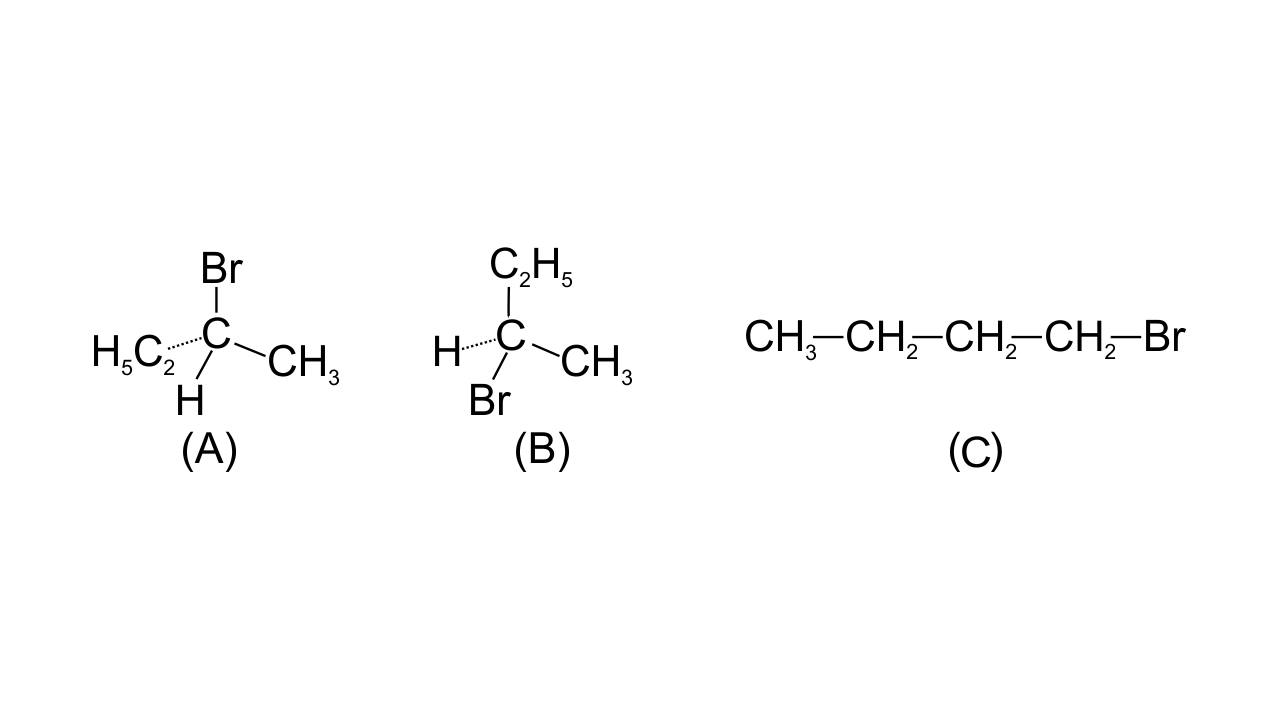The mixture consists of

(i) A and B as major and C as minor products

(ii) B as major, A and C as minor products

(iii) B as minor, A and C as major products

(iv) A and B as minor and C as major products

Ans: (i)

The reaction follows Markovnikov’s rule leading to giving 2o carbocation as an intermediate.

6. Which of the following will not show geometrical isomerism?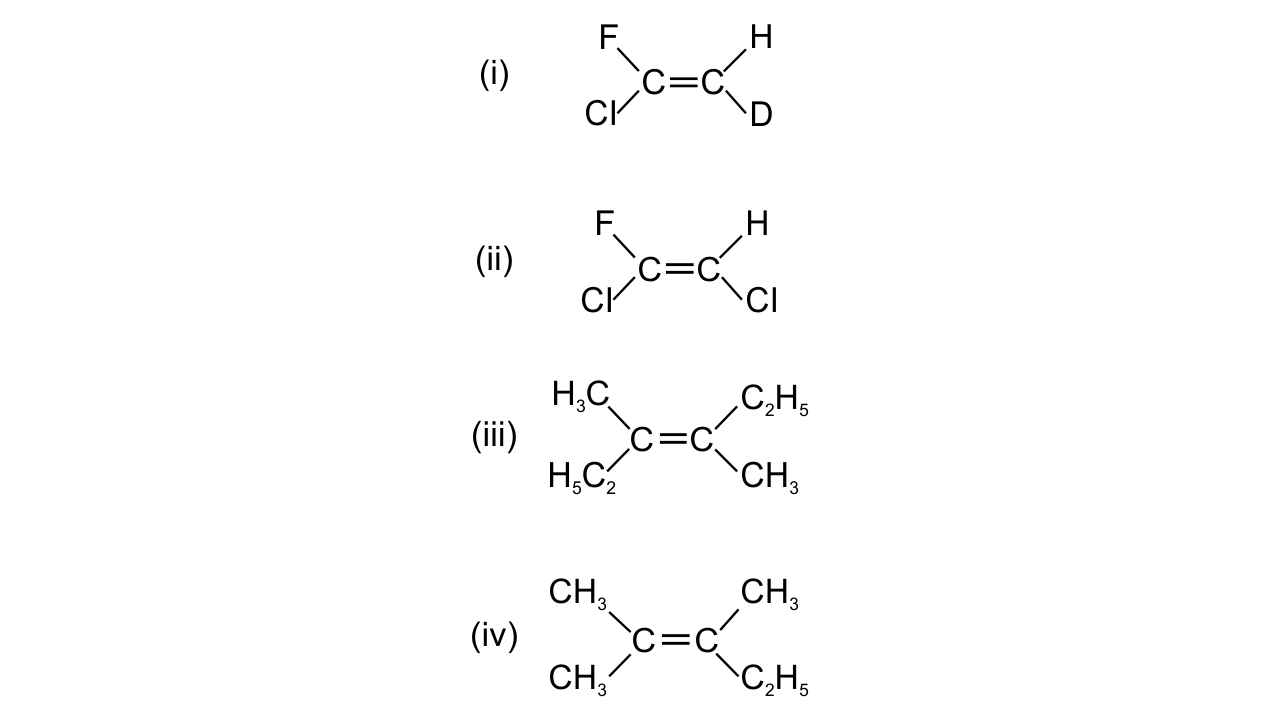Ans: (iv)

The two substituents attached to the same C atom of the C=C bond should be different to exhibit geometrical isomerisms.

7. Arrange the following hydrogen halides in order of their decreasing reactivity with propene.

(i) HCl>HBr> HI

(ii) HBr> HI >HCl

(iii) HI >HBr>HCl

(iv) HCl> HI >HBr

Ans: (iii)

The addition of alkyl halides to propene depends upon the H-X bond strength i.e. lesser the bond strength higher will be the reactivity of the alkyl halide. As down the group size of the halogens increases thus the bond strength decreases.

8. Arrange the following carbanions in order of their decreasing stability.

(A) H3C - C ≡ C-

(B) H - C ≡ C-

(C) H3C - CH2

(i) A > B > C

(ii) B > A > C

(iii) C > B > A

(iv) C > A > B

Ans: (ii)

The electronegativity of sp hybridization is higher than that of sp3 hybridization.

The +I effect destabilizes the carbanion.

9. Arrange the following alkyl halides in decreasing order of the rate of β– elimination reaction with alcoholic KOH.(i) A > B > C

(ii) C > B > A

(iii) B > C > A

(iv) A> C > B

Ans: (iv)

The order of reactivity of β– elimination reaction is 3o>2o>1o

10. Which of the following reactions of methane is incomplete combustion:

(i) 2CH4 + O2 $\xrightarrow{Cu/523k/100atm}$ 2CH3OH

(ii) CH4 + O2 $\xrightarrow{M_20_3}$ HCHO +  H2O

(iii) CH4 + O2 → C (s) +  2H2O (l)

(iv) CH4 + O2 CO2 (g) +  2H2O (l)

Ans: (iii)

As the unburn carbon left out in this reaction thus it is an incomplete reaction

### Multiple Choice Questions (Type-II)

11. Some oxidation reactions of methane are given below. Which of them is/are controlled oxidation reactions?

(i) CH4 + O2 CO2 (g) +  2H2O (l)

(ii) CH4 + O2 C (s) +  2H2O (l)

(iii) CH4 + O2 $\xrightarrow{M_20_3}$ HCHO +  H2O

(iv) 2CH4 + O2 $\xrightarrow{Cu/523k/100atm}$ 2CH3OH

Ans: (iii) & (iv)

Methane is a controlled oxidation reaction that leads to giving formaldehyde and methanol.

12. Which of the following alkenes on ozonolysis give a mixture of ketones only?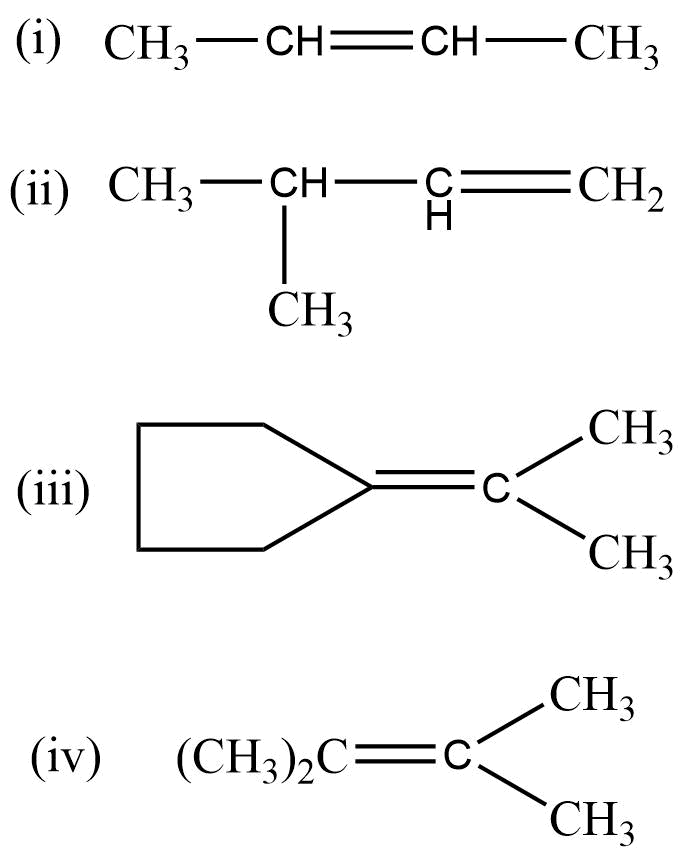Ans: (iii) & (iv)

When only the alkyl group is attached to the C atom of the C=C bond then only it would lead to giving only ketone on ozonolysis.

13. Which are the correct IUPAC names of the following compound?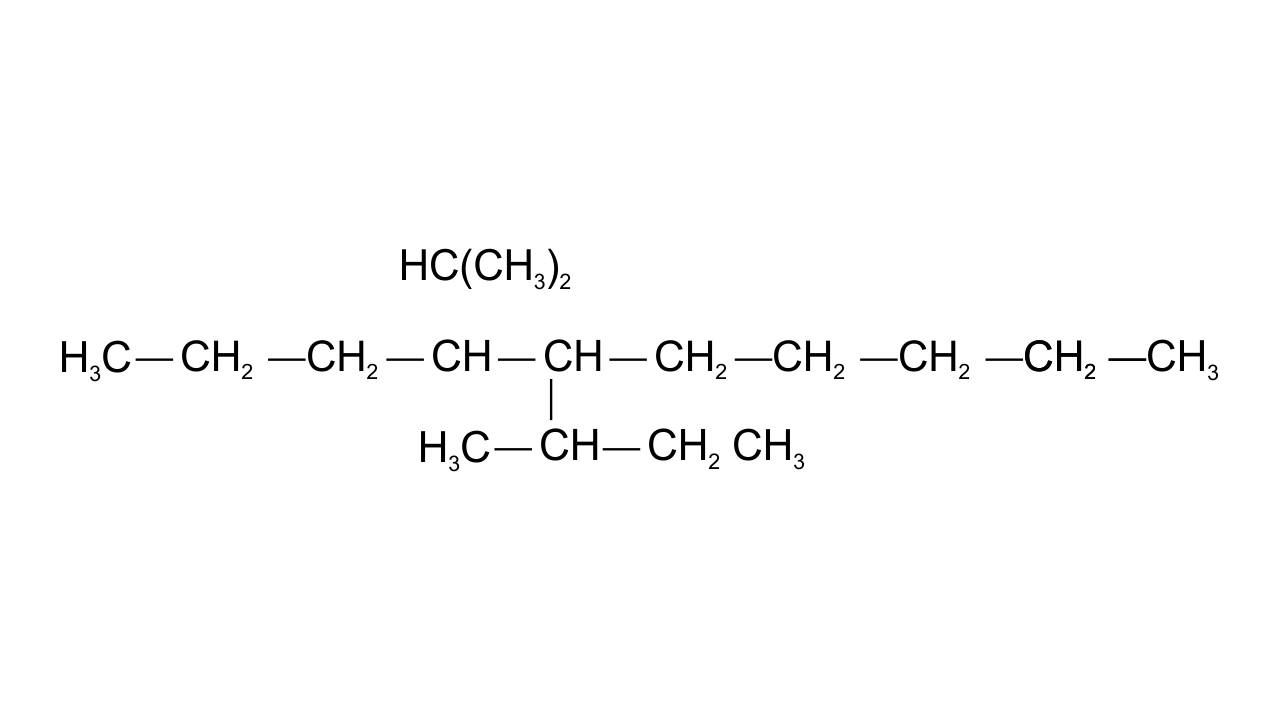(i) 5– Butyl – 4– isopropyldecane

(ii) 5– Ethyl – 4– propyldecane

(iii) 5– sec-Butyl – 4– iso-propyldecane

(iv) 4–(1-methylethyl)– 5 – (1-methylpropyl)-decane

Ans:(iii) & (iv)

The longest carbon chain is of 10 carbon atom

14. Which are the correct IUPAC names of the following compound?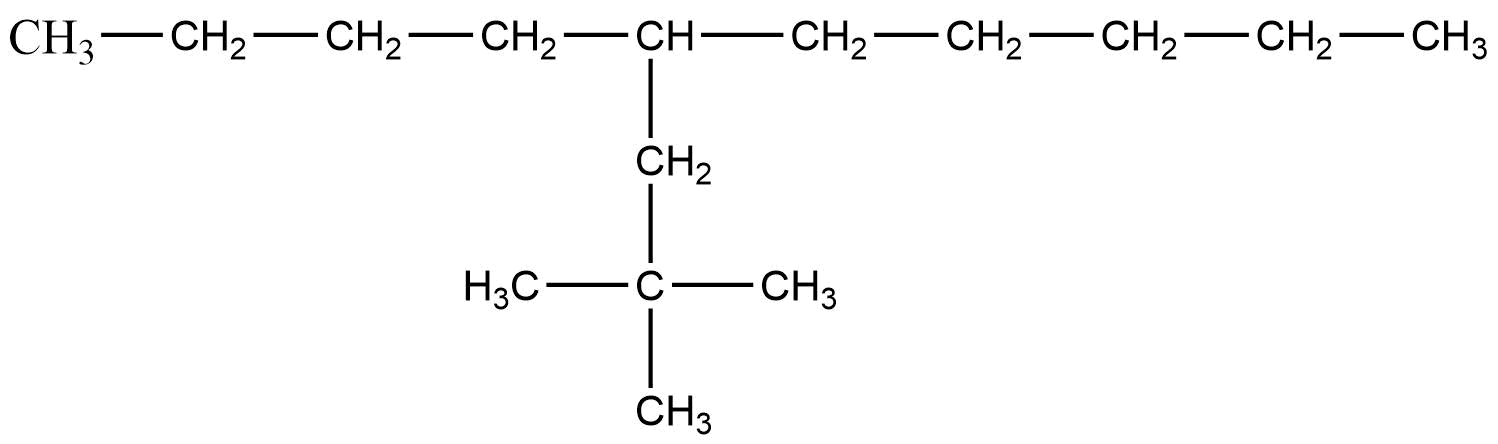Ans: (i) & (iv)

The longest carbon chain is of 10 carbon atom

15. For an electrophilic substitution reaction, the presence of a halogen atom in the benzene ring _______.

(i) deactivates the ring by inductive effect

(ii) deactivates the ring by resonance

(iii) increases the charge density at ortho and para position relative to meta position by resonance

(iv) directs the incoming electrophile to meta position by increasing the charge density relative to ortho and para position.

Ans: (i) & (iii)

The halogens atom weakly deactivate the ring due to the -I effect and directs the incoming group to the ortho and para position due to the +R effect.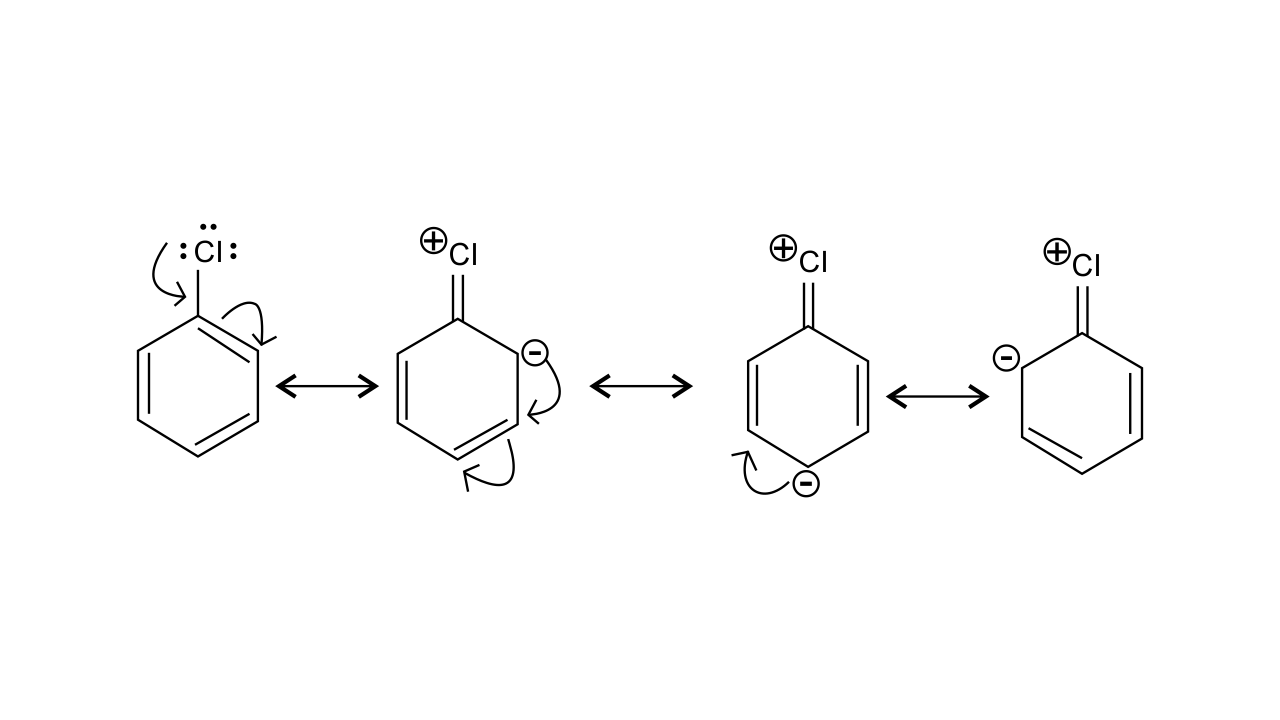16. In an electrophilic substitution reaction of nitrobenzene, the presence of nitro group ________.

(i) deactivates the ring by inductive effect.

(ii) activates the ring by inductive effect.

(iii) decreases the charge density at ortho and para position of the ring relative to meta position by resonance.

(iv) increases the charge density at meta position relative to the ortho and para positions of the ring by resonance

Ans: (i) & (iii)

The nitro group strongly deactivate the ring due to the -R effect and -I effect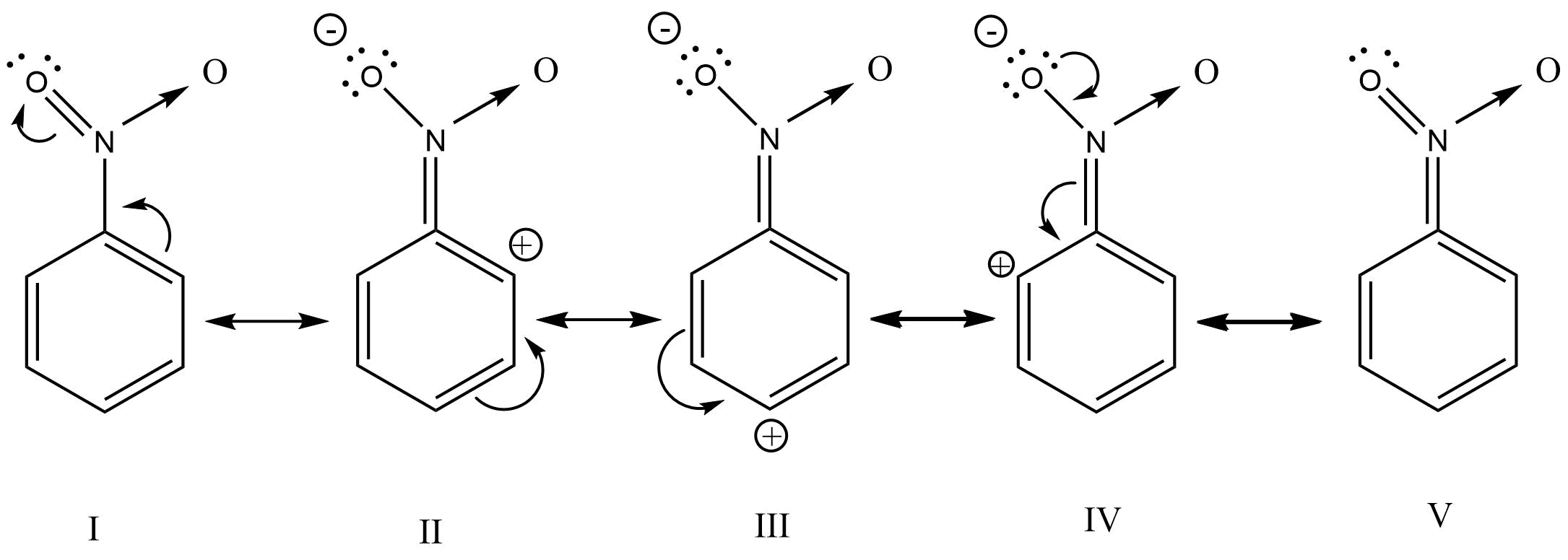17. Which of the following are correct?

(i) CH3—O—CH2 is more stable than CH3—CH2

(ii) (CH3)2CH is less stable than CH3—CH2—CH2

(iii) CH2=CH—CH2 is more stable than CH3—CH2—CH2

(iv) CH2=CHis more stable than CH3—CH2

Ans: (i) & (iii)

The +I effect of the -OCH3 group stabilizes the carbocation.

The two resonating structure of CH2=CH-CH2CH2-CH=CH2 makes it more stable

18. Four structures are given in options (i) to (iv). Examine them and select the aromatic structures.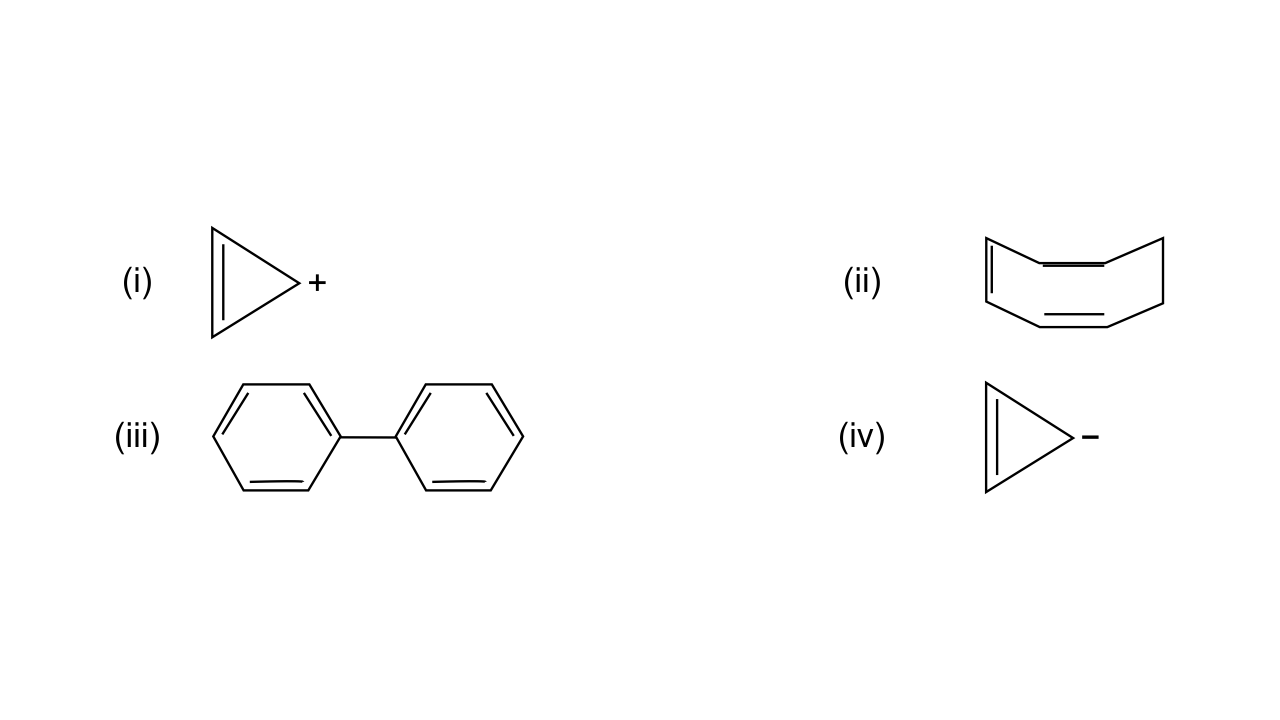Ans: (i) & (iii)

The structures that follow the (4n+2) Huckle rule of aromaticity is considered to be aromatic

19. The molecules having dipole moment are __________.

(i) 2,2-Dimethylpropane

(ii) trans-Pent-2-ene

(iii) cis-Hex-3-ene

(iv) 2, 2, 3, 3 - Tetramethylbutane.

Ans: (ii) & (iii)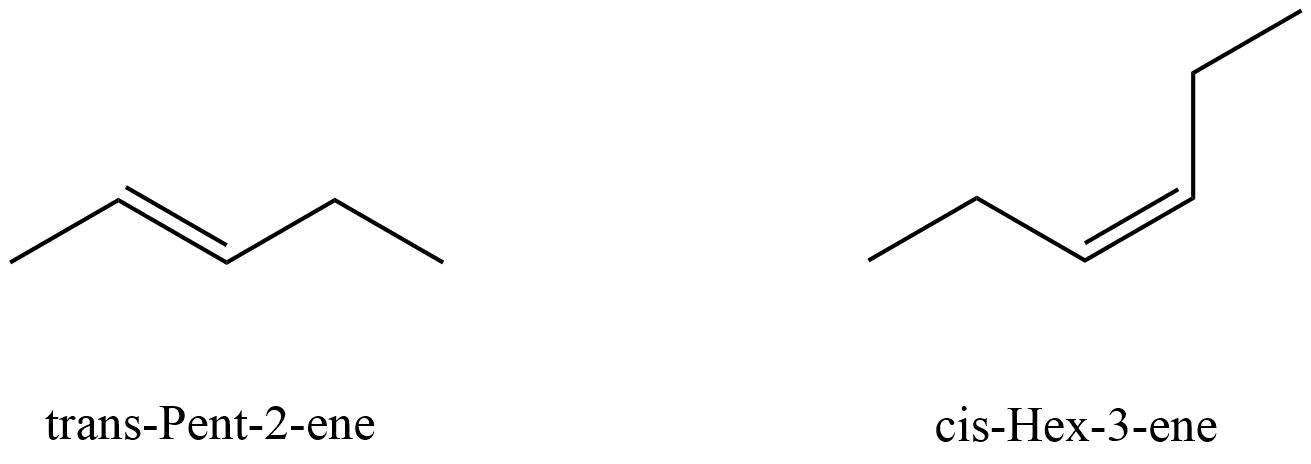20. Why do alkenes prefer to undergo electrophilic addition reactions while arenes prefer electrophilic substitution reactions? Explain.

Ans: If arenes would undergo electrophilic addition reaction then they would lose their aromaticity which would lead to comparatively less stability. However, alkenes undergo electrophilic addition reaction via carbocation intermediate.

21. Alkynes on reduction with sodium in liquid ammonia form trans alkenes. Will the butene thus formed on reduction of 2-butyne show the geometrical isomerism?

Ans: Yes, it will execute geometrical isomerism as the product formed can be either cis-2-butene or trans-2-butene.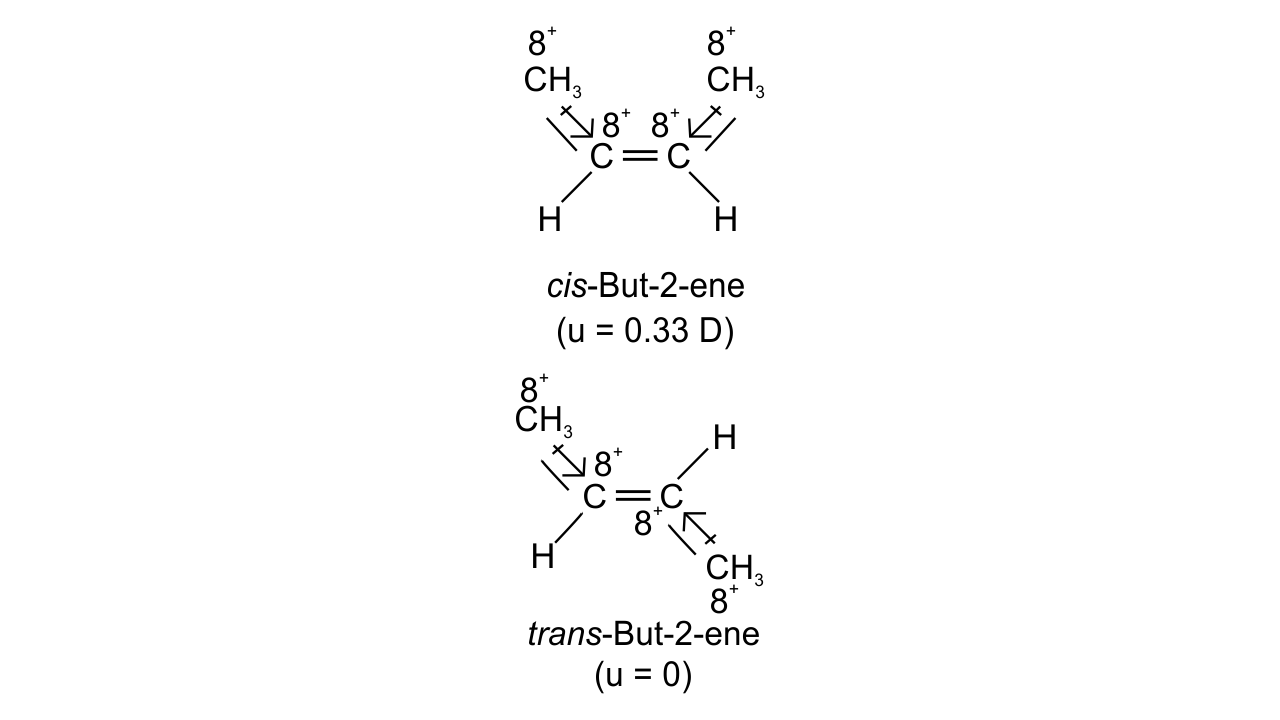22. Rotation around carbon-carbon single bond of ethane is not completely free. Justify the statement.

Ans: Therotation around C-C single bond is possible but it is not completely free due to the torsional strain which is about 1-20 KJ mol-1.

23. Draw Newman and Sawhorse projections for the eclipsed and staggered conformations of ethane. Which of these conformations is more stable and why?

Ans: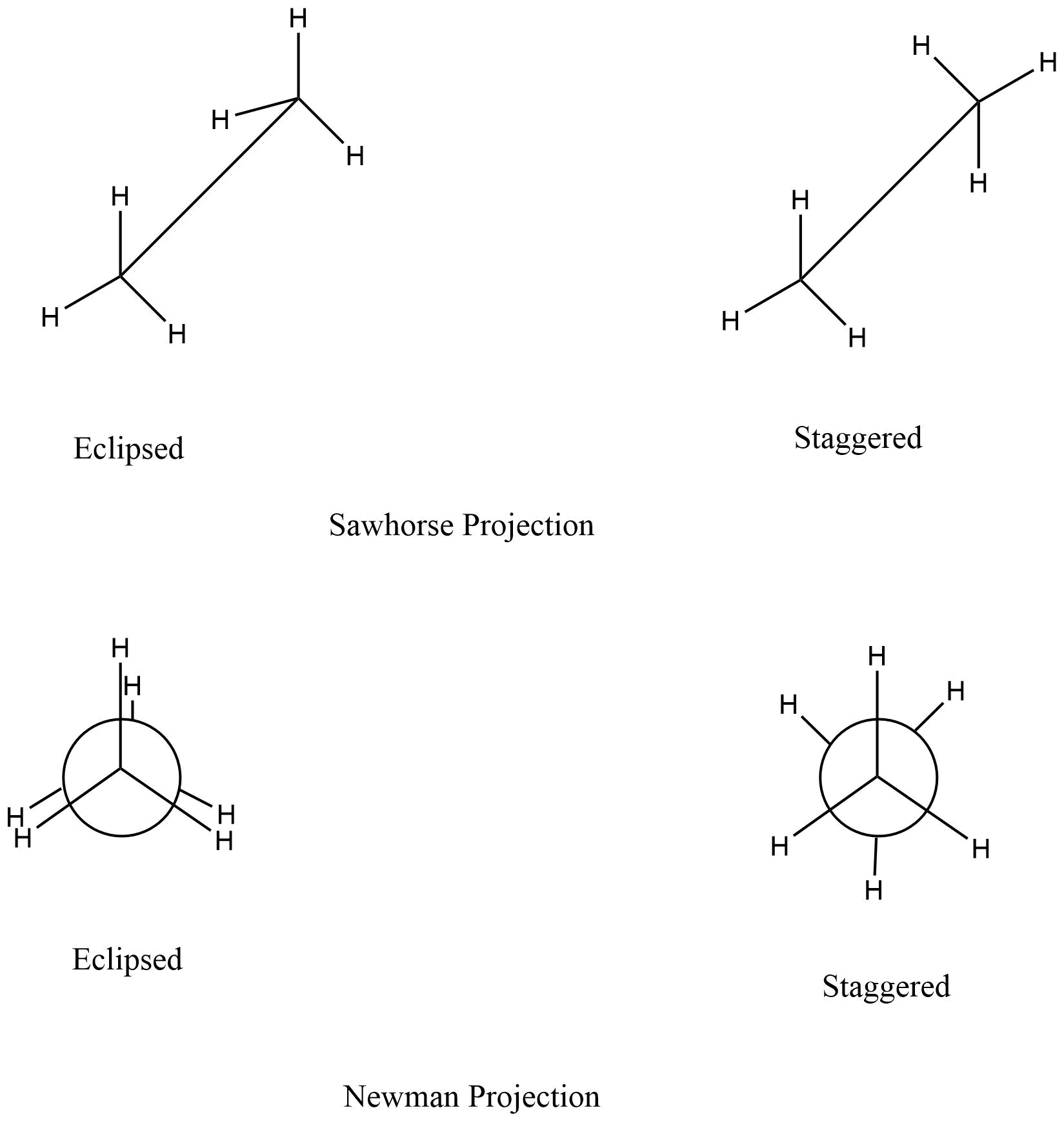The staggered conformation is more stable because of comparatively less torsional strain.

24. The intermediate carbocation formed in the reactions of HI, HBr and HCl with propene is the same and the bond energy of HCl, HBr and HI is 430.5 kJ mol–1, 363.7 kJ mol–1 and 296.8 kJ mol–1 respectively. What will be the order of reactivity of these halogen acids?

Ans: The rate-determining step involved in the reaction is

CH3-CH=CH2 + HX → CH3-CH+-CH3 + X-

So the rate-determining step depends on the bond energy of HX i.e. higher the bond energy lesser would be reactivity.

Thus the order of reactivity of these halogen acids is

HI>HBr>HCl

25. What will be the product obtained as a result of the following reaction and why?Ans: Thebenzene here would undergo Friedel-Crafts alkylation reaction where carbocation is formed as an intermediate, thus secondary carbocation is formed as an intermediate due to its greater stability than that of the primary carbocation.

The final product obtained is26. How will you convert benzene into

(i) p – nitrobromobenzene

(ii) m – nitrobromobenzene

Ans:

(i)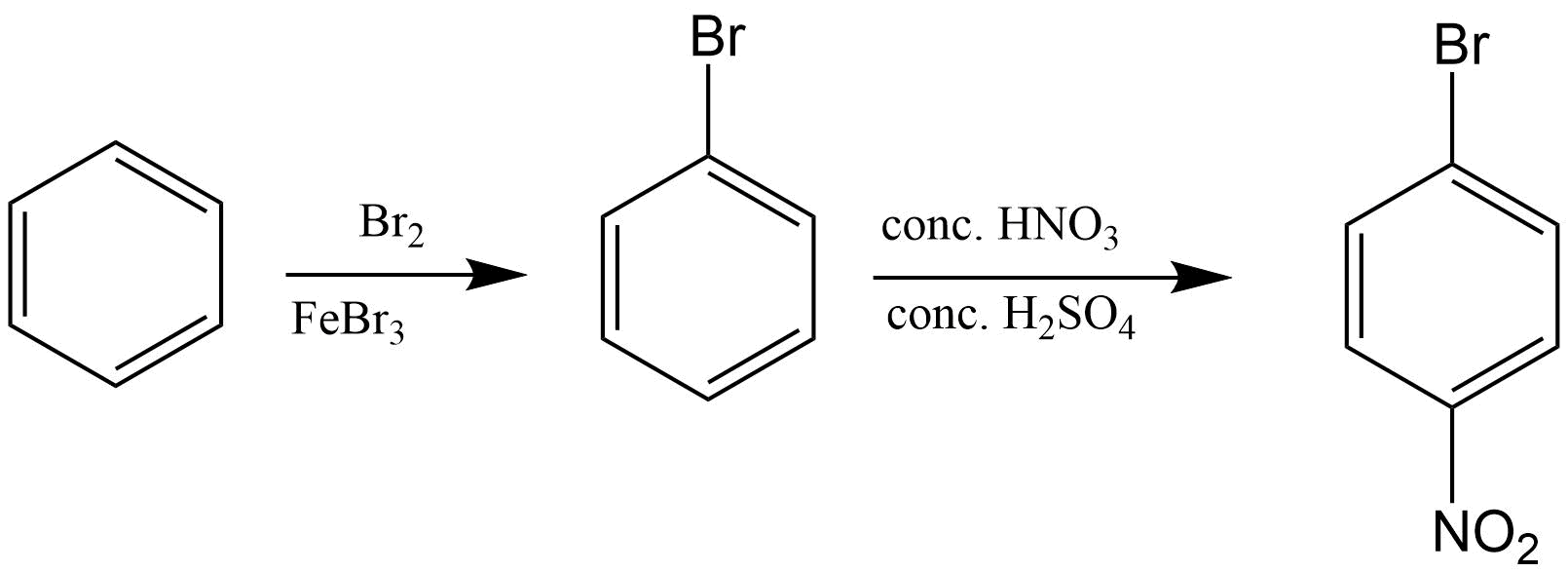(ii)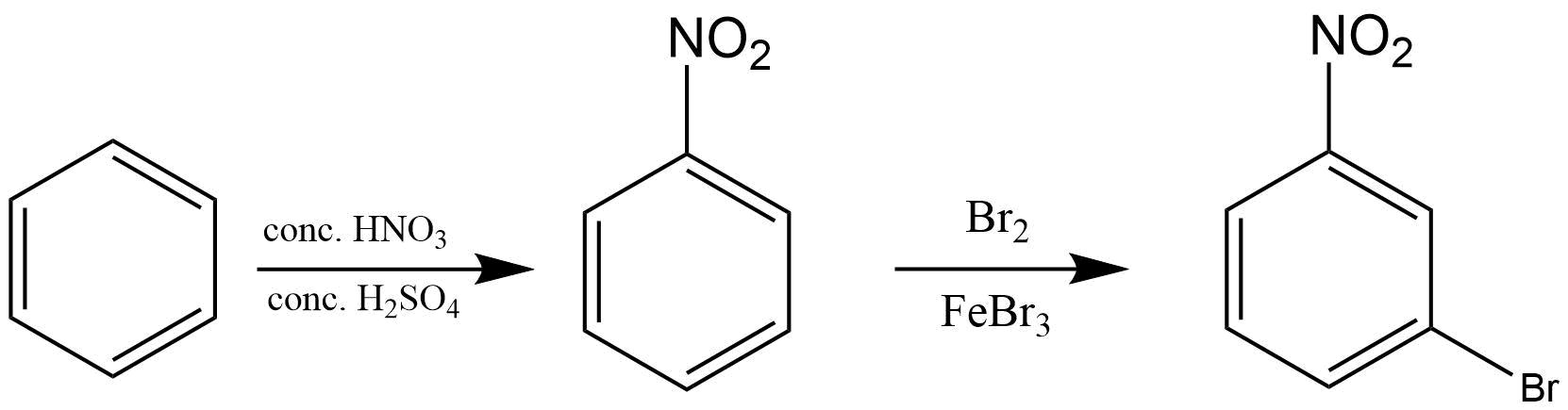27. Arrange the following set of compounds in the order of their decreasing relative reactivity with an electrophile.Give reason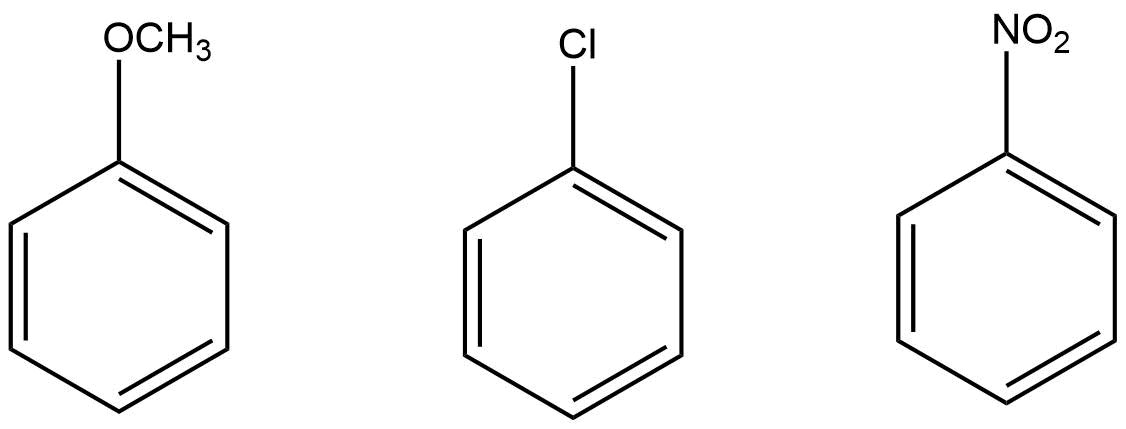Ans: The electron donating group increases the reactivity in electrophilic substitution reaction whereas the electron withdrawing group decreases the reactivity in electrophilic substitution.

Thus, the order of reactivity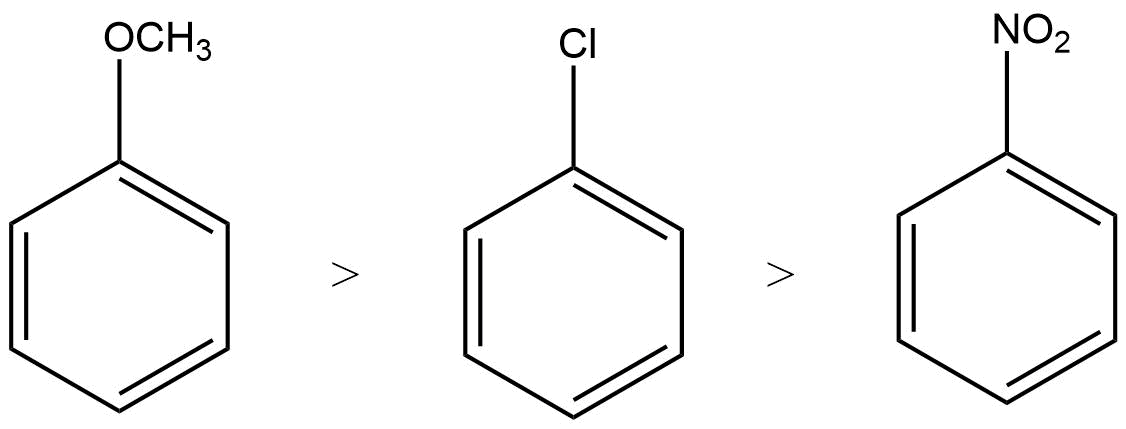28. Despite their - I effect, halogens are o- and p-directing in haloarenes. Explain.

Ans: Due to the +R effect halogens are ortho and para directing groups.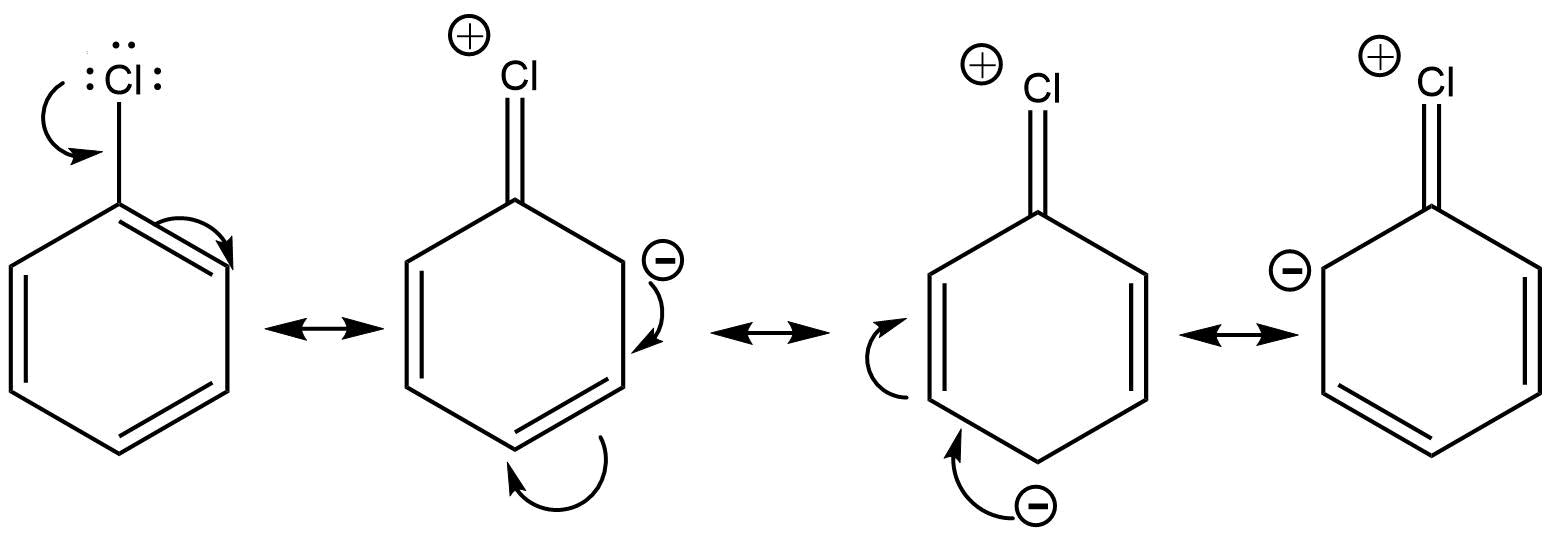29. Why does presence of a nitro group make the benzene ring less reactive in comparison to the unsubstituted benzene ring. Explain.

Ans: The nitro group strongly deactivate the benzene towards the electrophilic substitution reaction due to the -R and -I effect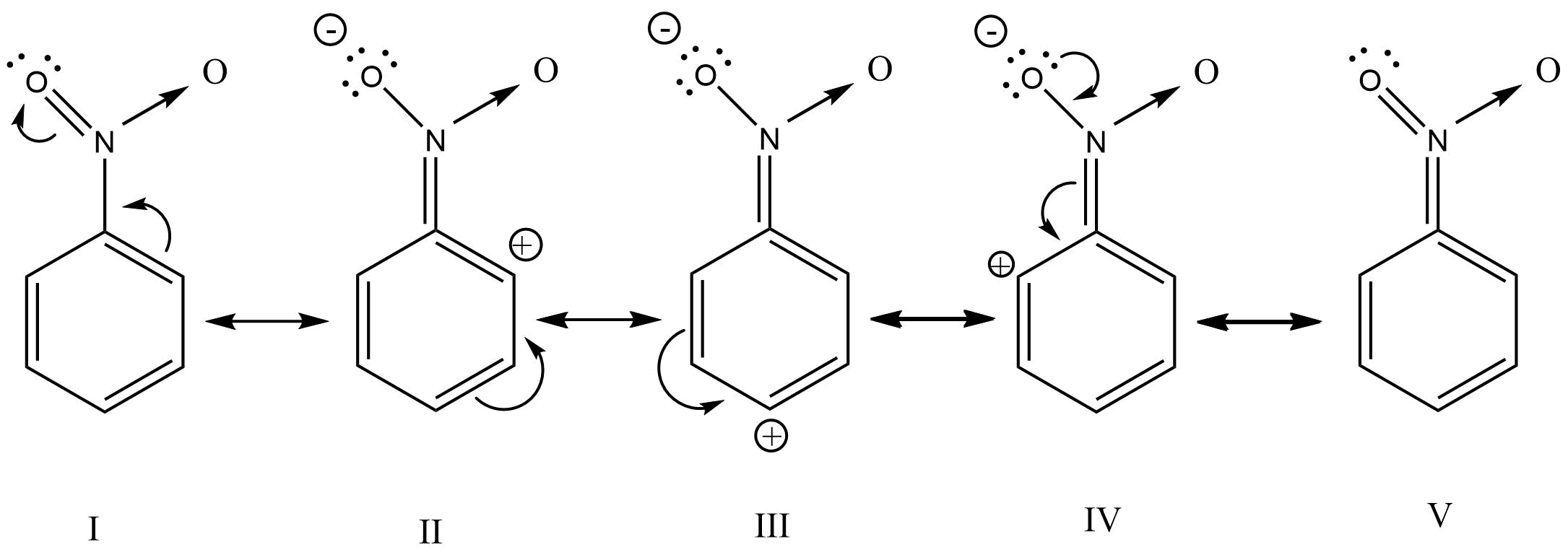30. Suggest a route for the preparation of nitrobenzene starting from acetylene?

Ans: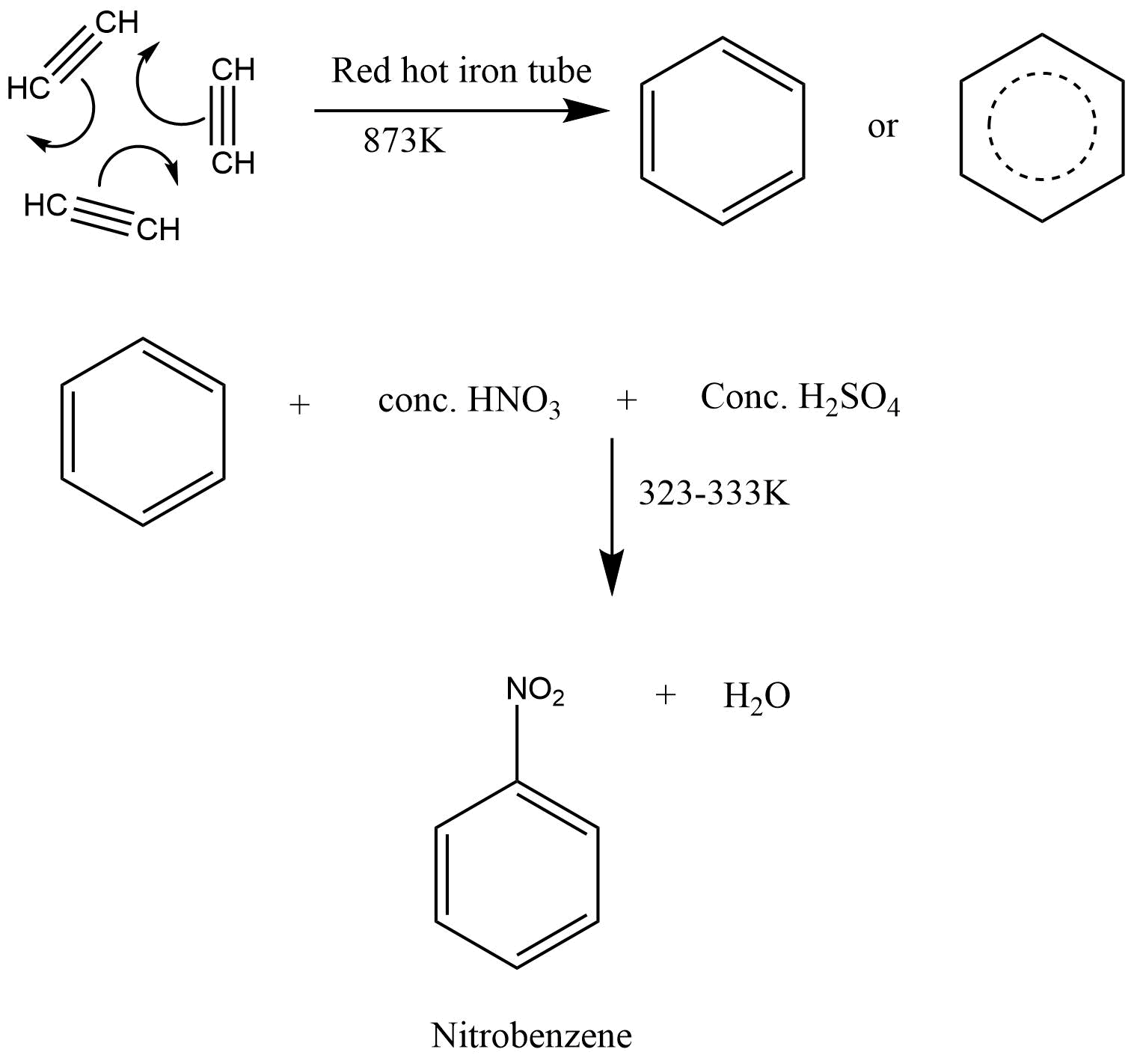31. Predict the major product (s) of the following reactions and explain their formation.Ans: In presence of (Ph-CO-O)2it lead to CH3-CH2-CH2Br as the reaction undergo by free radical mechanism

However in absence of (Ph-CO-O)2 the reaction undergo by carbocation intermediate thus lead to give CH3-CHBr-CH3

32. Nucleophiles and electrophiles are reaction intermediates having electron rich and electron deficient centres respectively. Hence, they tend to attack electron deficient and electron rich centres respectively. Classify the following species as electrophiles and nucleophiles.

(i) H3CO-

(ii)(iii)(iv)(v) (H3C)3C+

(vi) Br-

(vii) H3COH

(viii) R-NH-R

Ans:

 Electrophiles Nucleophiles (iii),(iv),(v) (i),(ii),(vi),(vii),(viii)

33. The relative reactivity of 1°, 2°, 3° hydrogen’s towards chlorination is 1 : 3.8 : 5. Calculate the percentages of all monochlorinated products obtained from 2-methylbutane.

Ans:  The structure of 2-methylbutane is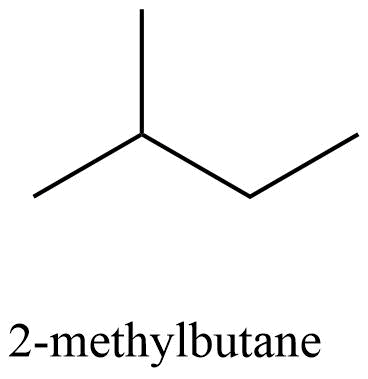Total number Total amount of monochlorinatedProduct % of monochlorinated product 10 9 9 41.7 20 2 7.6 35.2 30 1 5 23.1

34. Write the structures and names of products obtained in the reactions of sodium with a mixture of 1-iodo-2-methylpropane and 2-iodopropane.

Ans: It is a Wurtzreaction where alkane is formed.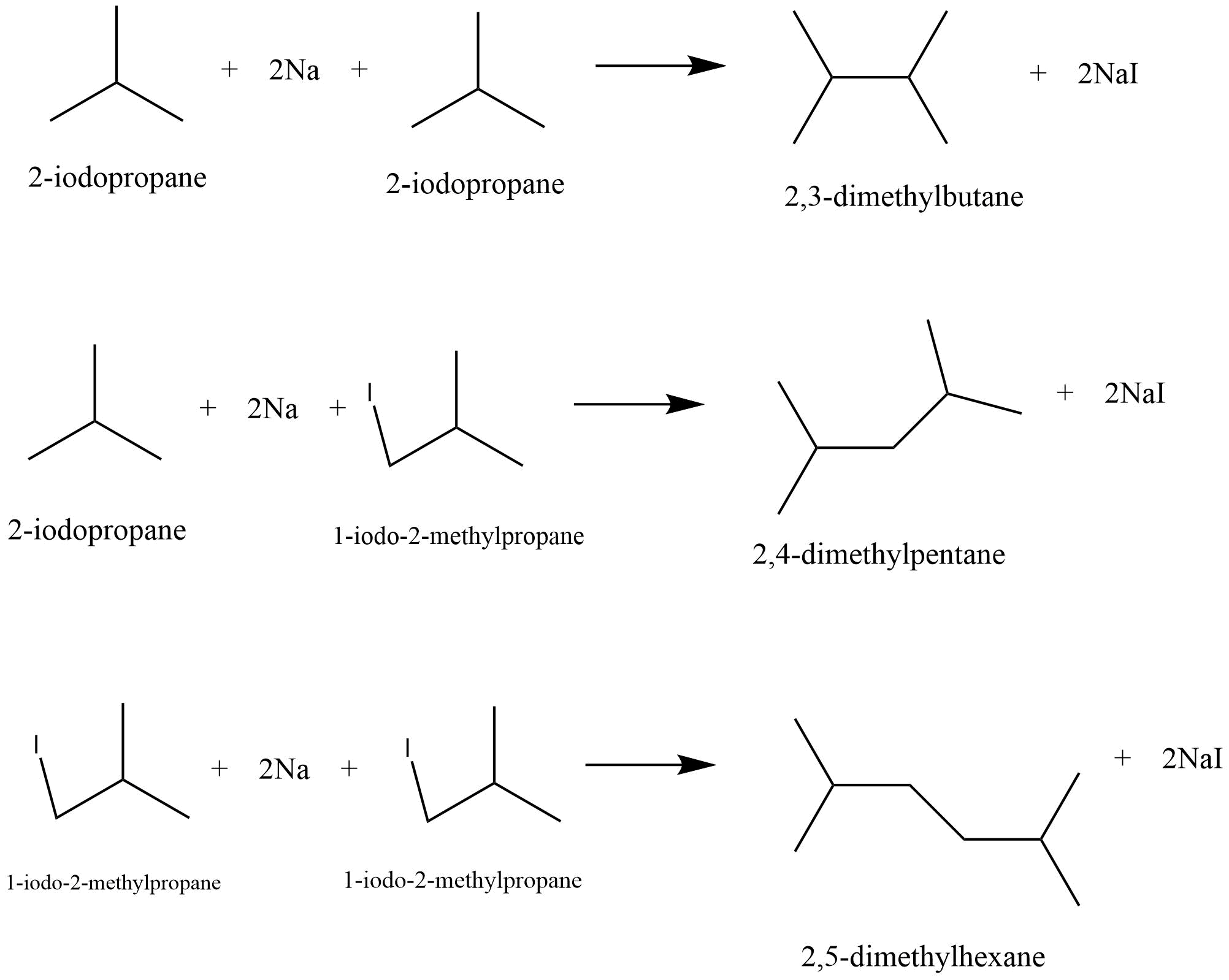35. Write hydrocarbon radicals that can be formed as intermediates during monochlorination of 2-methylpropane? Which of them is more stable? Give reasons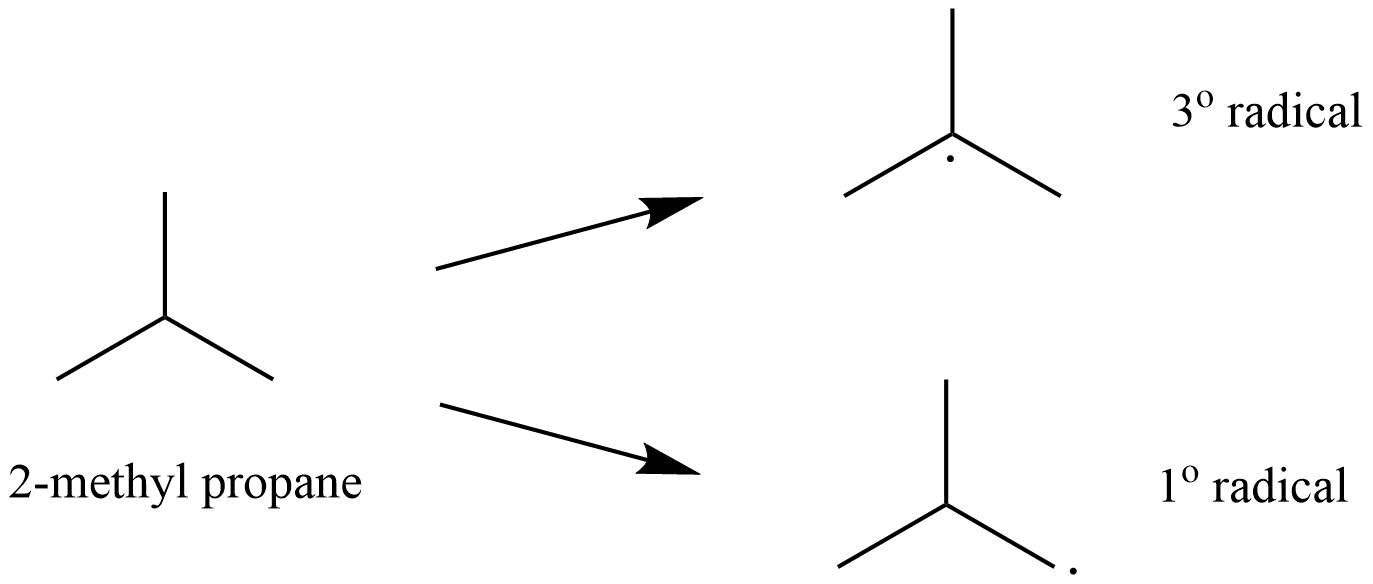Among the two 3o radical is most stable as it contains 9 ∝ -hydrogen whereas 1o radical contains only 1 ∝ -hydrogen.

36. An alkane C8H18 is obtained as the only product on subjecting a primary alkyl halide to Wurtz reaction. On monobromination this alkane yields a single isomer of a tertiary bromide. Write the structure of alkane and the tertiary bromide.

Ans: The reaction is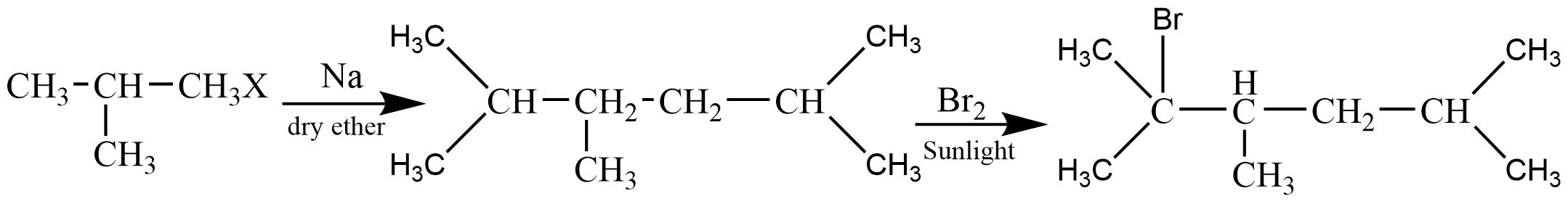The alkane is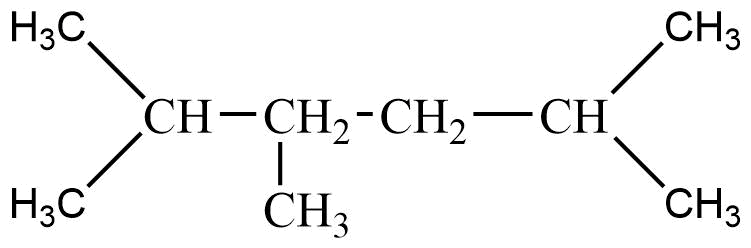The tertiary bromide is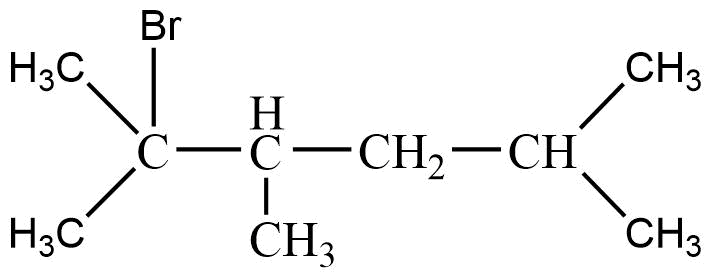37. The ring systems having following characteristics are aromatic.

(i) Planar ring containing conjugated π bonds.

(ii) Complete delocalisation of the π−electrons in ring system i.e. each atom in the ring hasunhybridised p-orbital, and

(iii) Presence of (4n+2) π−electrons in the ring where n is an integer (n = 0, 1, 2,...........) [Huckel rule].

Using this information classify the following compounds as aromatic/nonaromatic.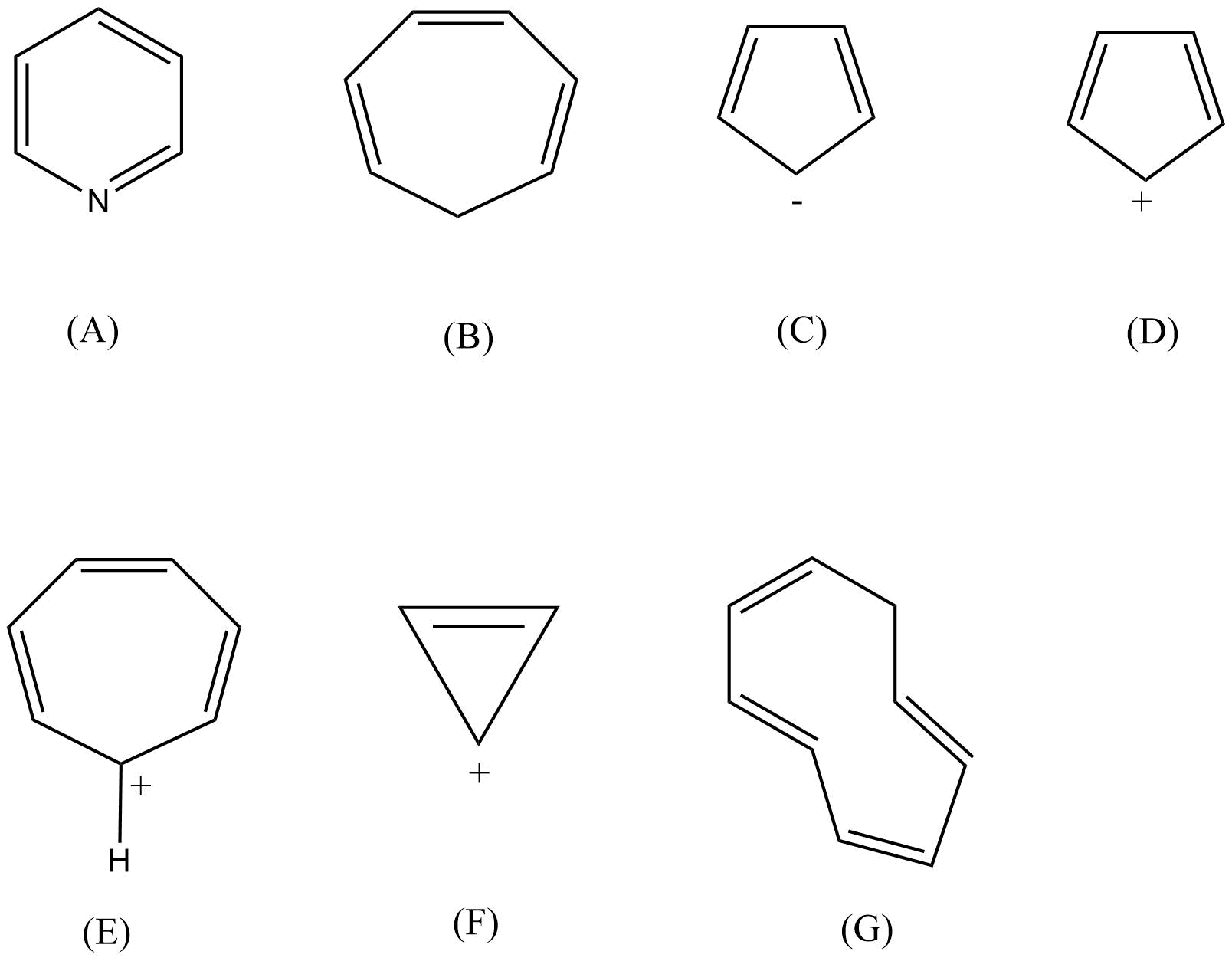Ans:

 Aromatic Non-aromatic A,C,E,F B,D,G

38. Which of the following compounds are aromatic according to Huckel’s rule?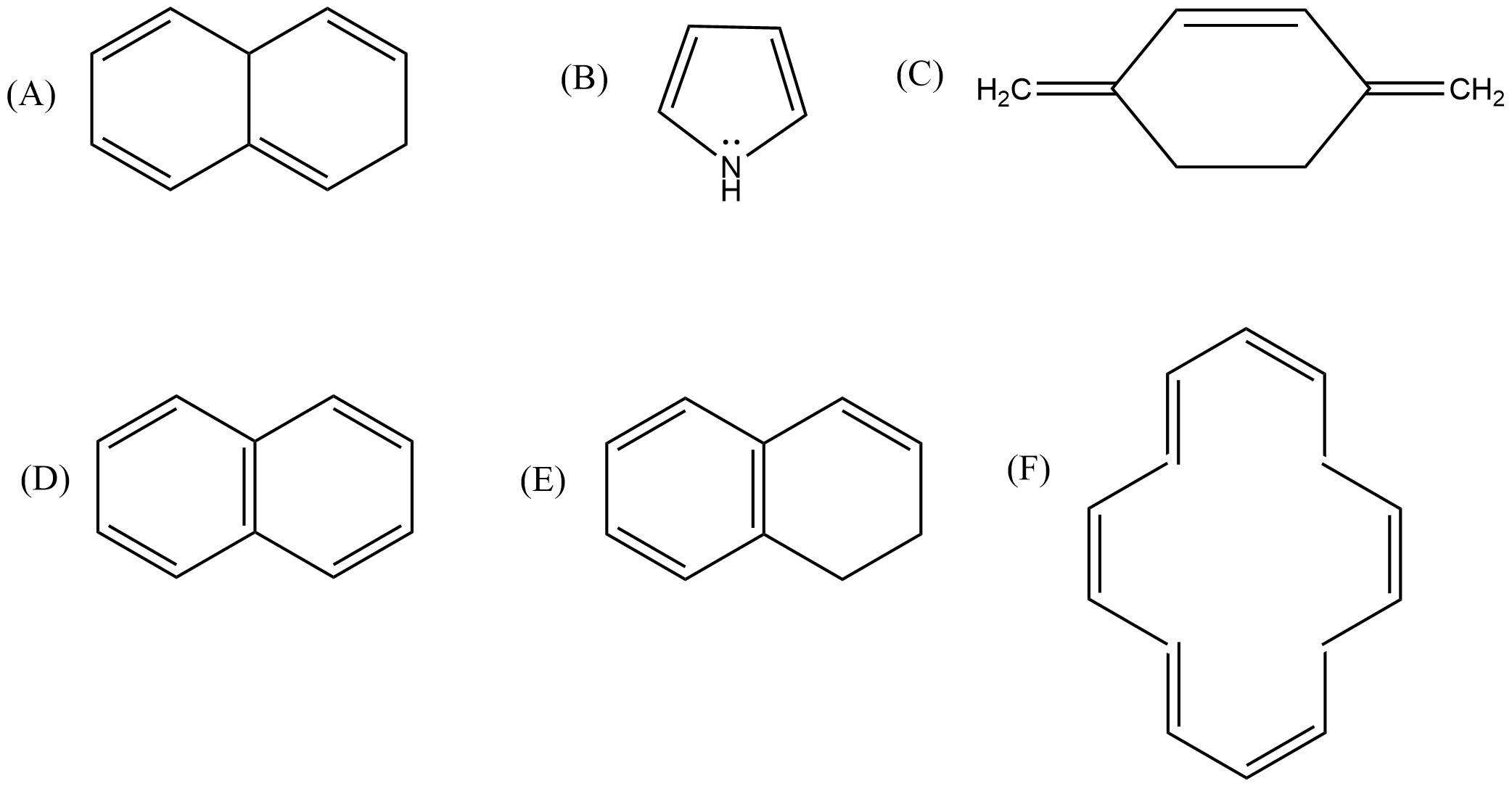Ans: As per the Huckel (4n+2) rule following distinction can be made:-

 Aromatic Non aromatic B,D,F A,C,E

39. Suggest a route to prepare ethyl hydrogensulphate (CH3–CH2–OSO2—OH) starting from ethanol (C2H5OH).

Ans: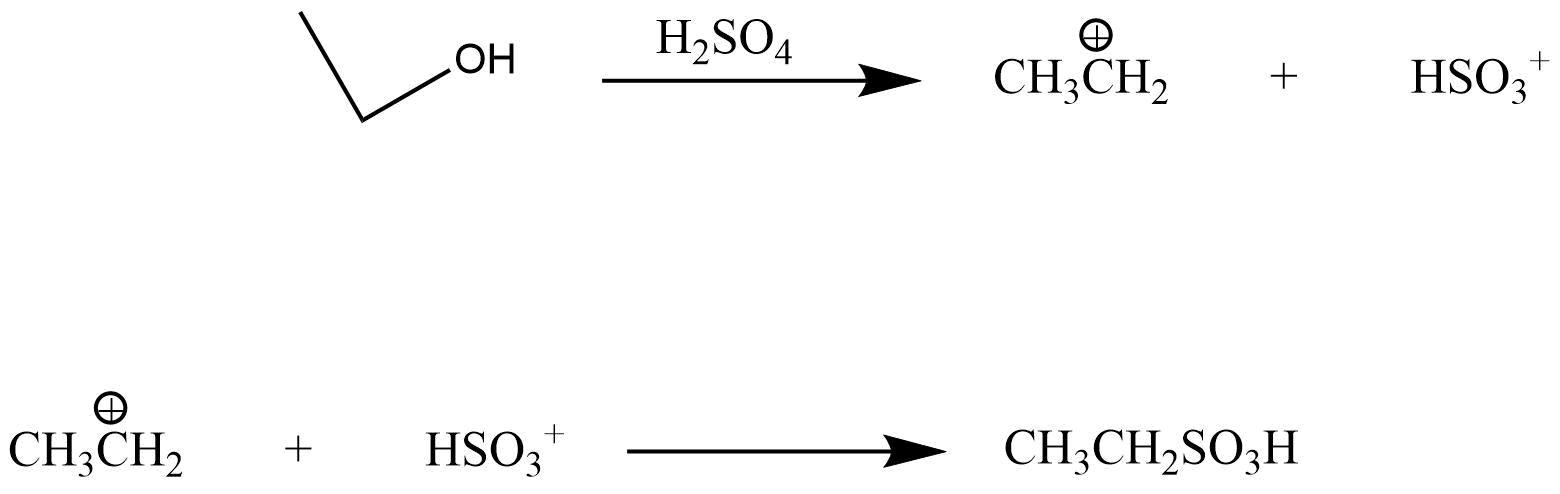### Matching Type

40. Match the reagent from Column I which on reaction with

CH3 – CH=CH2 gives some product given in Column II as per the codes given below:

 Column I Column II (i) O3/Zn + H2O (a) Acetic acid and CO2 (ii) KMnO4/H+ (b) Propan-1-ol (iii) KMnO4/OH- (c) Propan-2-ol (iv) H2O/H+ (d) Acetaldehyde and formaldehyde (v) B2H6/NaOH and H2O2 (e) Propan-1,2-diol

Ans:

 (i) O3/Zn + H2O (d) Acetaldehyde and formaldehyde (ii) KMnO4/H+ (a)Acetic acid and CO2 (iii) KMnO4/OH- (e) Propan-1,2-diol (iv) H2O/H+ (c) Propan-2-ol (v) B2H6/NaOH and H2O2 (b) Propan-1-ol

41. Match the hydrocarbons in Column I with the boiling points given in Column II.

 Column I Column II (i) n-pentane (a) 282.5 K (ii) iso-pentane (b) 309 K (iii) neo-pentane (c) 301 K

Ans:

 (i) n-pentane (b) 309 K (ii) iso-pentane (c) 301 K (iii) neo-pentane (a) 282.5 K

42. Match the following reactants in Column I with the corresponding reaction products in Column II.

 Column I Column II (i) Benzene + Cl2$\xrightarrow{AlCl_3}$ (a) Benzoic acid (ii)Benzene + CH3Cl $\xrightarrow{AlCl_3}$ (b) Methyl phenyl ketone (iii)Benzene + CH3COCl $\xrightarrow{AlCl_3}$ (c) Toluene (iv)Toluene $\xrightarrow{KMno_{4}/NaOH}$ (d) Chlorobenzene (e) Benzene hexachloride

Ans:

 (i) Benzene + Cl2 $\xrightarrow{AlCl_3}$ (d) Chlorobenzene (ii)Benzene + CH3Cl $\xrightarrow{AlCl_3}$ (c) Toluene (iii)Benzene + CH3COCl $\xrightarrow{AlCl_3}$ (b) Methyl phenyl ketone (iv)Toluene $\xrightarrow{KMno_{4}/NaOH}$ (a) Benzoic acid

43. Match the reactions given in Column I with the reaction types in Column II.

 Column I Column II (i) CH2=CH2 + H2O $\xrightarrow{H^{+}}$ CH3CH2OH (a) Hydrogenation (ii) CH2 ≡  CH2 + H2 $\xrightarrow{Pd}$ CH3CH3 (b) Halogenation (iii) CH2 ≡ CH2 + Cl2 → ClCH2CH2Cl (c) Polymerisation (iv) 3CH ≡ CH $\xrightarrow[\text{tube heat}]{Cu}$C6H6 (d) Hydration (e) Condensation

Ans:

 (i) CH2=CH2 + H2O $\xrightarrow{H^{+}}$ CH3CH2OH (d) Hydration (ii) CH2=CH2 + H2 $\xrightarrow{Pd}$ CH3CH3 (a) Hydrogenation (iii) CH2=CH2 + Cl2→ClCH2CH2Cl (b) Halogenation (iv) 3CH ≡ CH $\xrightarrow[\text{tube heat}]{Cu}$ C6H6 (c) Polymerisation

### Assertion and Reason Type

In the following questions a statement of assertion (A) followed by a statement of reason (R) is given. Choose the correct option out of the choices given below each question.

44. Assertion (A): The compound cyclooctane has the following structural formula:It is cyclic and has conjugated 8π-electron system but it is not an aromatic compound.

Reason (R): (4n + 2) π electrons rule does not hold good and ring is not planar.

(i) Both A and R are correct and R is the correct explanation of A.

(ii) Both A and R are correct but R is not the correct explanation of A.

(iii) Both A and R are not correct.

(iv) A is not correct but R is correct.

Ans: (i)

Because cyclooctatetraene is tubbed shaped.

45. Assertion (A): Toluene on Friedal Crafts methylation gives o– and p–xylene.

Reason (R): CH3-group bonded to benzene ring increases electron density at o– and p– position.

(i) Both A and R are correct and R is the correct explanation of A.

(ii) Both A and R are correct but R is not the correct explanation of A.

(iii) Both A and R are not correct.

(iv) A is not correct but R is correct.

Ans:

(i) The -CH3 group is an activating group and it directs the upcoming substituent to the ortho and para position.

46. Assertion (A): Nitration of benzene with nitric acid requires the use of concentrated sulphuric acid.

Reason (R): The mixture of concentrated sulphuric acid and concentrated nitric acid produces the electrophile, NO2 +

(i) Both A and R are correct and R is the correct explanation of A.

(ii) Both A and R are correct but R is not the correct explanation of A.

(iii) Both A and R are not correct.

(iv) A is not correct but R is correct.

Ans: (i)

The sulphuric acid helps in furnishing NO2+(electrophile).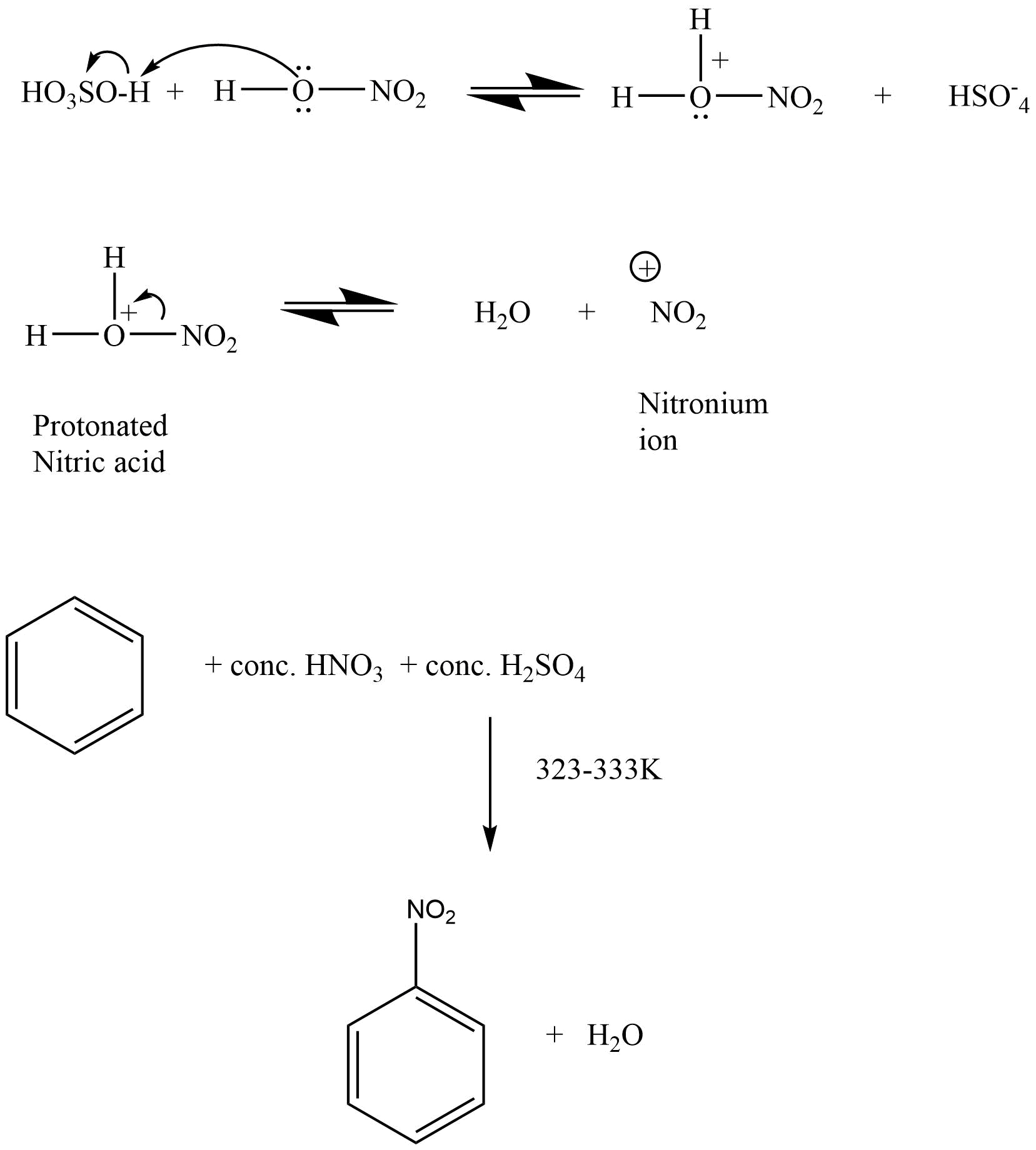47. Assertion (A): Among isomeric pentanes, 2, 2-dimethylpentane has highest boiling point.

Reason (R): Branching does not affect the boiling point.

(i) Both A and R are correct and R is the correct explanation of A.

(ii) Both A and R are correct but R is not the correct explanation of A.

(iii) Both A and R are not correct.

(iv) A is not correct but R is correct.

Ans:(iii)

With the branching the boiling decreases due to the decrease in surface area which leads to decrease its van der Waals force.

48. An alkyl halide C5H11Br (A) reacts with ethanolic KOH to give an alkene ‘B’, which reacts with Br2 to give a compound ‘C’, which on dehydrobromination gives an alkyne ‘D’. On treatment with sodium metal in liquid ammonia one mole of ‘D’ gives one mole of the sodium salt of ‘D’ and half a mole of hydrogen gas. Complete hydrogenation of ‘D’ yields a straight chain alkane. Identify A,B, C and D. Give the reactions invovled.

Ans: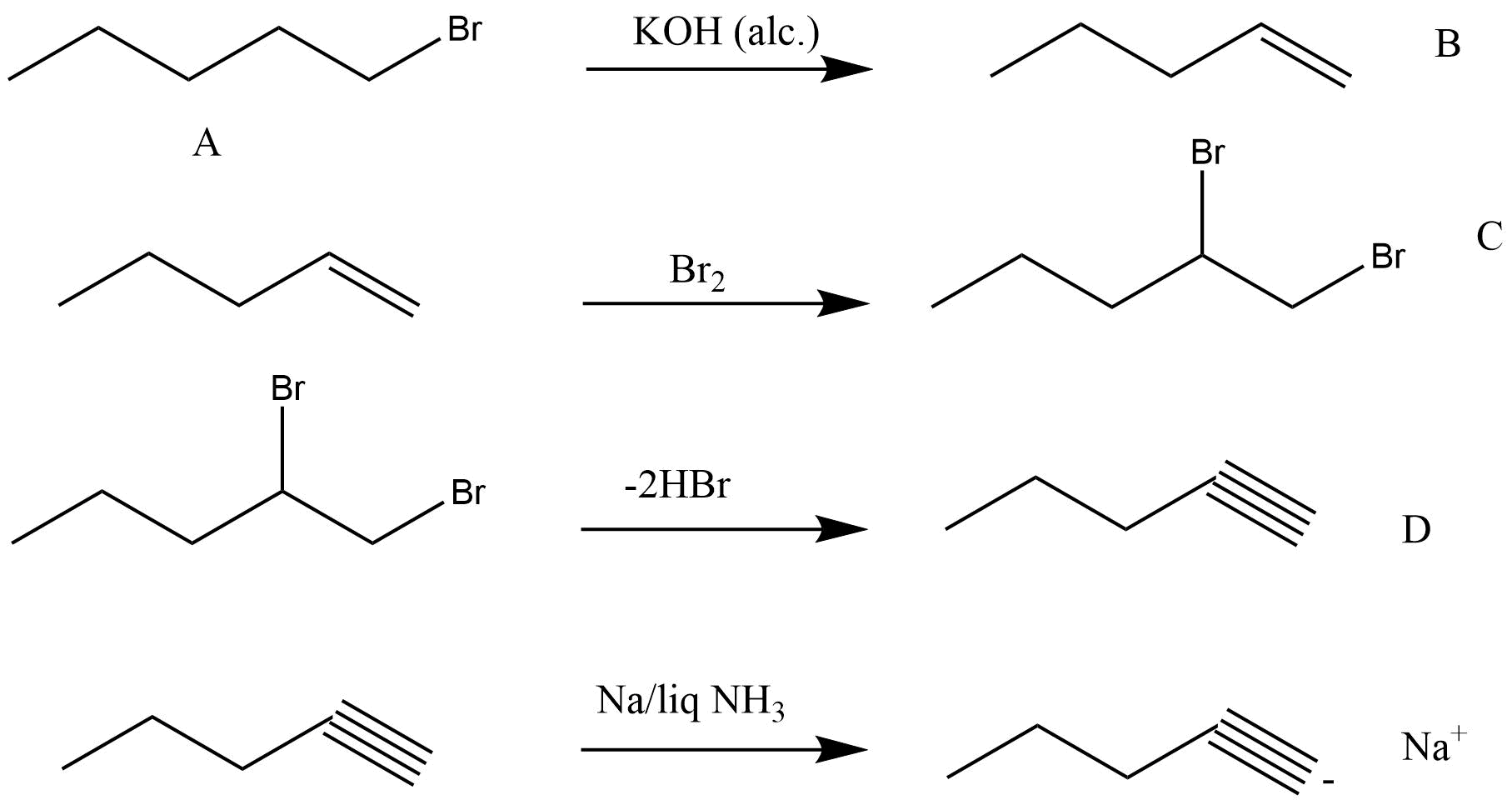49. 896 mL vapour of a hydrocarbon ‘A’ having carbon 87.80% and hydrogen 12.19% weighs 3.28g at STP. Hydrogenation of ‘A’ gives 2-methylpentane. Also ‘A’ on hydration in the presence of H2SO4 and HgSO4 gives a ketone ‘B’ having molecular formula C6H12O. The ketone ‘B’ gives a positive iodoform test. Find the structure of ‘A’ and give the reactions involved.

Ans: The molar mass of the compound = $\frac {3.28 \times 22400}{896}$ = 831gmol-1

 Element Percentage At.mass Relative ratio Simplest ratio C 87.8% 12 7.31 3 H 12.19% 1 12.19 5

Thus the empirical formula of the compound is C3H5

Molecular formula =n x empirical formula = (831/41) x C3H5 = C6H10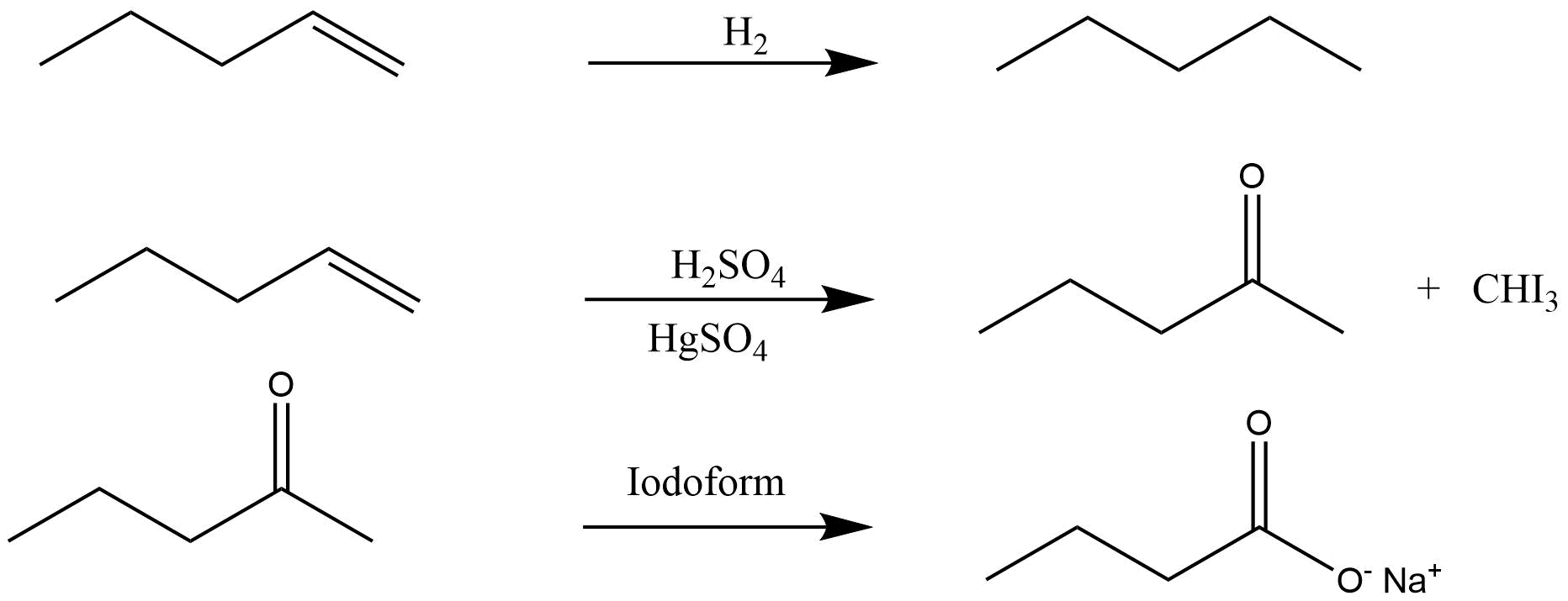50. An unsaturated hydrocarbon ‘A’ adds two molecules of H2 and on reductive ozonolysis gives butane-1,4-dial, ethanal and propanone. Give the structure of ‘A’, write its IUPAC name and explain the reactions involved.

Ans: The structure of A is

The reaction involved are as follows: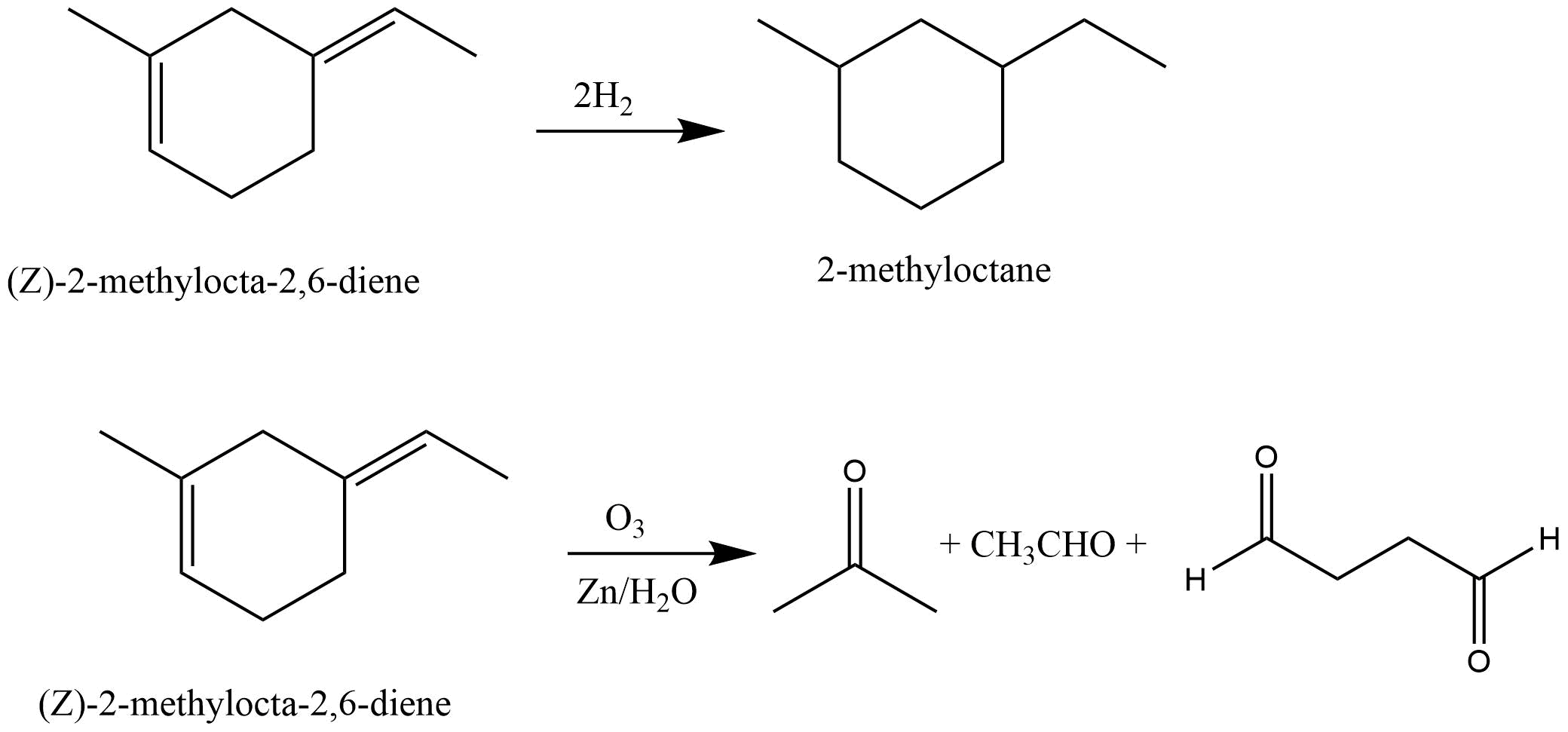51. In the presence of peroxide addition of HBr to propene takes place according to anti Markovnikov’s rule but peroxide effect is not seen in the case of HCl and HI. Explain.

Ans: The bond energy of HCl is higher than that of HBr thus it is not cleaved by free radical mechanism to exhibit peroxide effect. However in case of HI the bond energy is so low that the iodine radical forms readily and after formation it combines to form an iodine molecule.

### Summary of Chapter 13 Hydrocarbons

The compounds which contain only carbon and hydrogen are called hydrocarbons, all other families of organic compounds can be considered as derived from hydrocarbons by replacing one or more hydrogen atoms with other functional groups, which fits in well. Hydrocarbons naturally appear in our environment, our bodies are made up of many kinds of hydrocarbons.

Hydrocarbons play a very vital role in our daily lives. Till today, humans are highly dependent on hydrocarbons for their energy requirements, as they are a great source of energy. Most of the fuels used in energy generation in power plants and our houses are hydrocarbons. Petrol, coal, oil gas, kerosene, CNG (Compressed Natural Gas), LPG (Liquid natural gas), diesel etc are all hydrocarbons.

Hydrocarbons are also used in the manufacturing of polymers such as polythene, polypropylene, polystyrene etc. Most of the plastic may not look like it but is an example of hydrocarbon. Many kinds of life-saving drugs and dyes are manufactured using hydrocarbons, some hydrocarbons are also used as a solvent for paints.

Depending on the type of C-C bond present between them, they can be classified into these 3 categories:

• Saturated hydrocarbons

• Alkane

• Unsaturated hydrocarbons

• Alkene

• Alkyne

• Aromatic hydrocarbons

Chapter 13 covers all of these three types in a detailed manner. The student gets to know all about these hydrocarbons, they will also learn of the physical and chemical properties of these hydrocarbons, how they are prepared and how they react under different circumstances.

## FAQs on NCERT Exemplar for Class 11 Chemistry Chapter-13 (Book Solutions)

1. What are the major classification of Hydrocarbons according to chapter 13?

Compounds containing both carbons and hydrogens are linked to each other are called hydrocarbons. Depending on the type of carbon-carbon bond present in the hydrocarbon chain, they can be classified into three major categories-

1. Saturated hydrocarbons: These hydrocarbons contain only a C-C single bond. There are both open and closed chain hydrocarbons. Example -  Alkanes.

2. Unsaturated Hydrocarbons: They contain at least one C-C multiple bonds (double or triple bond). Depending upon the type of bond they can be further classified as Alkenes and Alkynes.

3. Aromatic hydrocarbons: Contains at least one special type of hexagonal ring of six carbon atoms with 3 C-C double bonds in alternate positions. An example will be benzene.

2. What are the necessary conditions for a hydrocarbon to be aromatic?

Aromatic hydrocarbons contain at least one special type of hexagonal ring of six carbon atoms with 3 C-C double bonds. Written below are some of the necessary conditions for a hydrocarbon to be aromatic-

1. Hydrocarbon should have a planar structure and should be cyclic.

2. The molecule of the hydrocarbon must contain a cloud of delocalized π-electrons above the ring.

3. For Aromaticity, The total number of π-electrons present in the ring should be equal to (4n+ 2), where n is an integer equal to 0, 1, 2 … etc. {Huckel’s rule}.

3. What are the properties of Benzene and its Homologues?

Following are the properties:

1. Benzene and its homologues, containing up to 8 carbons atoms, are colourless liquids with a distinctive smell, they appear as oils.

2. As aromatic hydrocarbons are like oils, they are immiscible in water but are soluble in organic solvents

3. They are very toxic and carcinogenic, which means they can cause cancer.

4. Benzene is also inflammable and burns with a yellowish sooty flame.

5. The melting and boiling points of aromatics substances like Benzene increases with the increase in molecular mass of the compound.

4. What are the major sub-topics from chapter 13 Hydrocarbons?

### Classification of Hydrocarbons:

• Alkanes

• Nomenclature And Isomerism of alkanes

• Preparation of alkanes

• Properties and conformations of alkanes

• Alkenes

• Structure Of Double Bond present in Alkenes

• Nomenclature and isomerism of alkenes

• Preparation and properties of it

• Alkynes

• Nomenclature And Isomerism of alkynes

• Structure Of Triple Bond present in Alkynes

• Preparation and properties of it

• Aromatic Hydrocarbon

• Nomenclature And Isomerism of Aromatic hydrocarbons

• Structure Of Benzene

• Aromaticity in hydrocarbons

• Preparation and Properties Of Benzene

• Carcinogenicity And Toxicity of various hydrocarbons.

5. What is meant by halogenations of Alkanes according to chapter 13 Hydrocarbon?

Halogenation is a type of substitution reaction in the replacement of one or more atoms (Commonly Hydrogen atoms) of alkanes by the corresponding number of Halogen atoms (Halogen are elements of group 17 of the periodic table). For example -

a. Chlorination of Alkanes: when the replacing halogen atom is of chlorine it is called Chlorination of alkanes. It is carried out by treating alkane with chlorine at the temperature of 523 - 773K or in the presence of sunlight.

CH4     +   Cl2 ⟶  CH3CL    +    HCL

b. Bromination of Alkanes: Bromination of alkanes works similarly but with bromine, and at a relatively slower pace.